# Ncert Class 6 Math Basic Geometrical Ideas Exercise 4.2

Basic Geometrical Ideas Class 6 Ex. 4.2

Ncert Class 6 Math Free Solution.

Exercise 4.2

Question 1 :- Classify the following curves as :
(i) open
(ii) closed.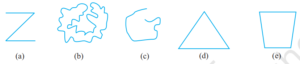Solution 1:-
(a) Open curve
(b) Closed curve
(c) Open curve
(d) Closed curve

Question 2 :- Draw rough diagrams to illustrate  following:
(a) Open curve
(b) Closed curve

Solution 2:-

(a)  Open curve(b) Closed curve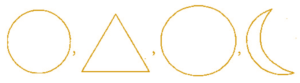Question 3 :- Draw any polygon and shade its interior?

Solution3 :-
ABCDE is the required polygon whose interior region is shaded.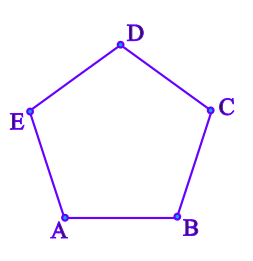Question 4 :- Consider the given figure and answer the questions.(a) Is it a curve?
(b) Is it closed?
Solution 4:-

(a) Yes, it is a curve.
(b) Yes, it is closed curve.

Question 5 :- Illustrate, if possible, each one of the following with  rough diagram :
(a) A closed curve that is not  polygon.
(b) An open curve made up entirely of the line segments.
(c) A polygon with two sides.

Solution 5:-

(a)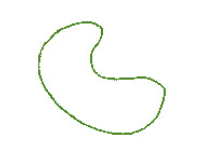(b)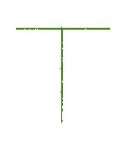(c) A polygon with two sides cannot be draw.